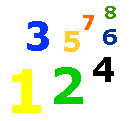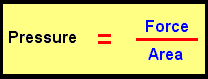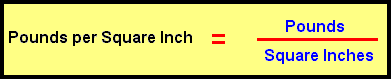# 4-12 Concept of Pressure

### Lesson List

Pressure is force per unit area.  The force of a book on a table is also pressure on the table.  The formula for pressure is:The pressure being exerted by the book on the table will be the pounds per square inch.  We will divide the pounds of force from the book by the number of square inches covered by the book.  A square inch is an area 1 inch by 1 inch.Let's say the book weights 2 pounds.  The area covered on the table by the book is 4 inches by 6 inches.  The squares inches would be 4 X 6 = 24 sq. in.

 Pressure    = 2 lb ------------ 24 sq in

The pressure of the book on the table is:

 2 1 of a pound per square inch ---- or ---- 24 12

Complete the questions below.

Pressure is per unit area.

The formula for pressure is force divided by .

Area is the number of inches.

A square inch is an area inch by inch.

An object that measures 8 inches by 10 inches will have an area of square inches.

If an object weighs 4 pounds and covers an area of 20 square inches, its pressure will be fifth of a pound per square inch.